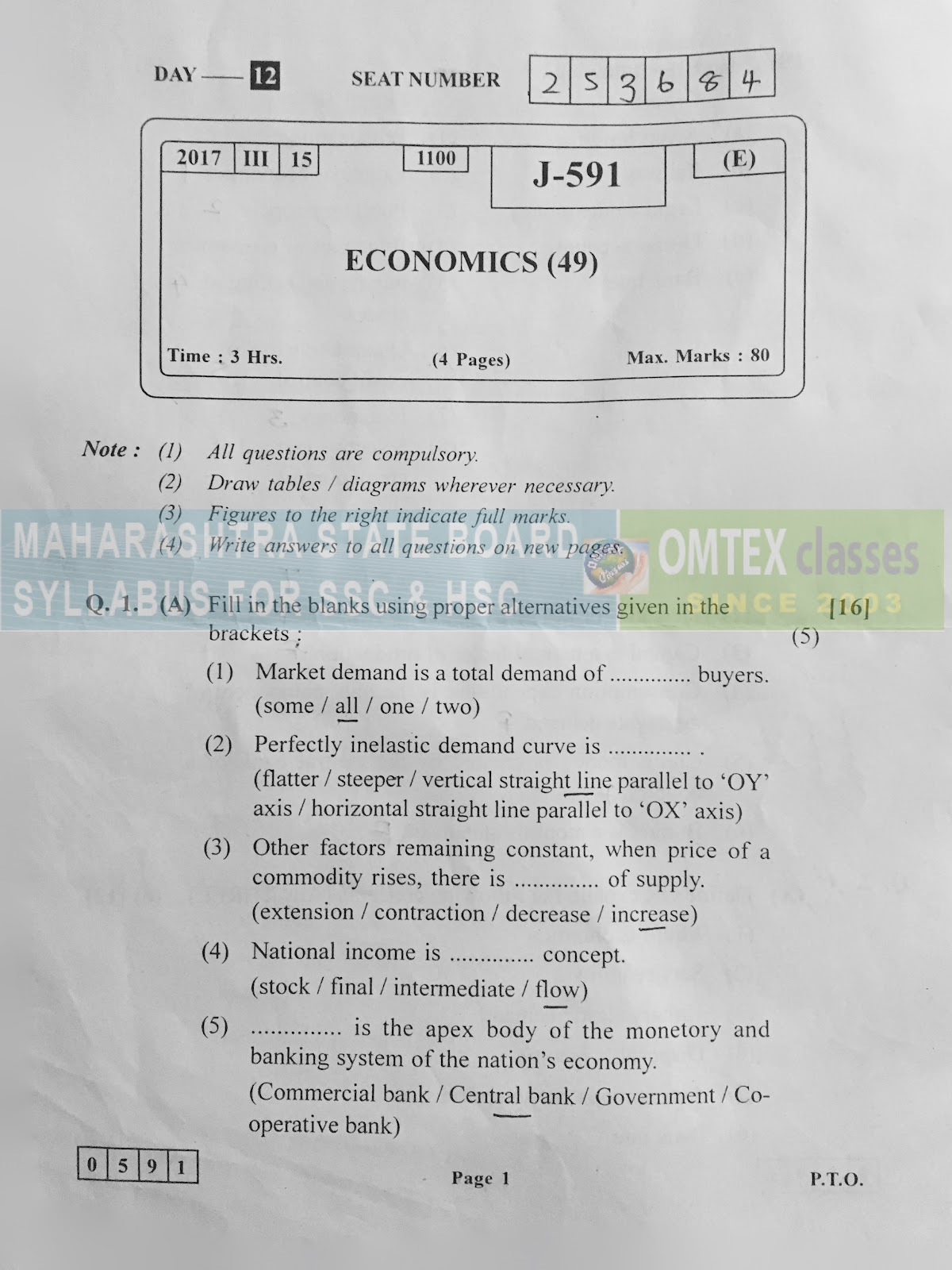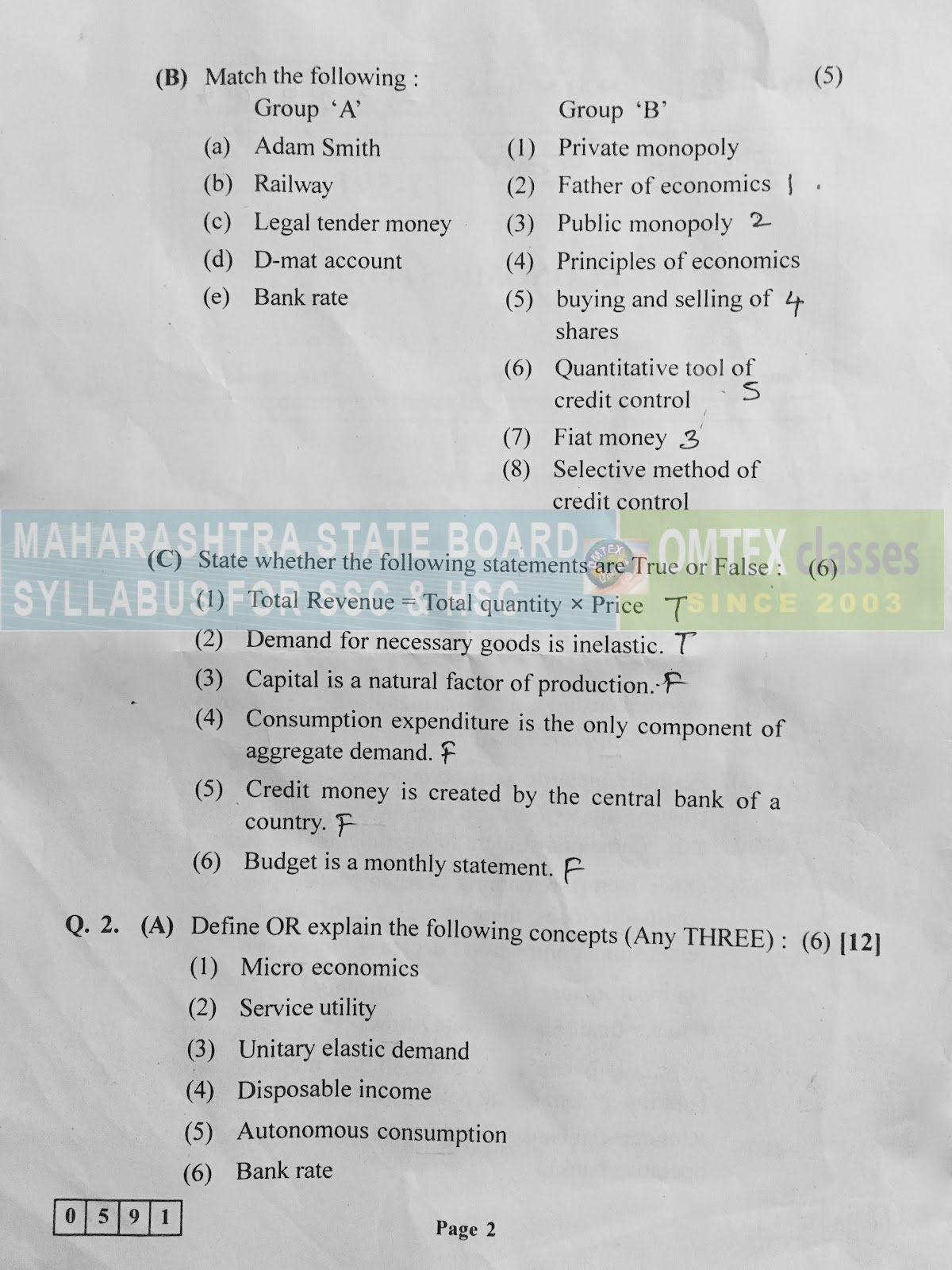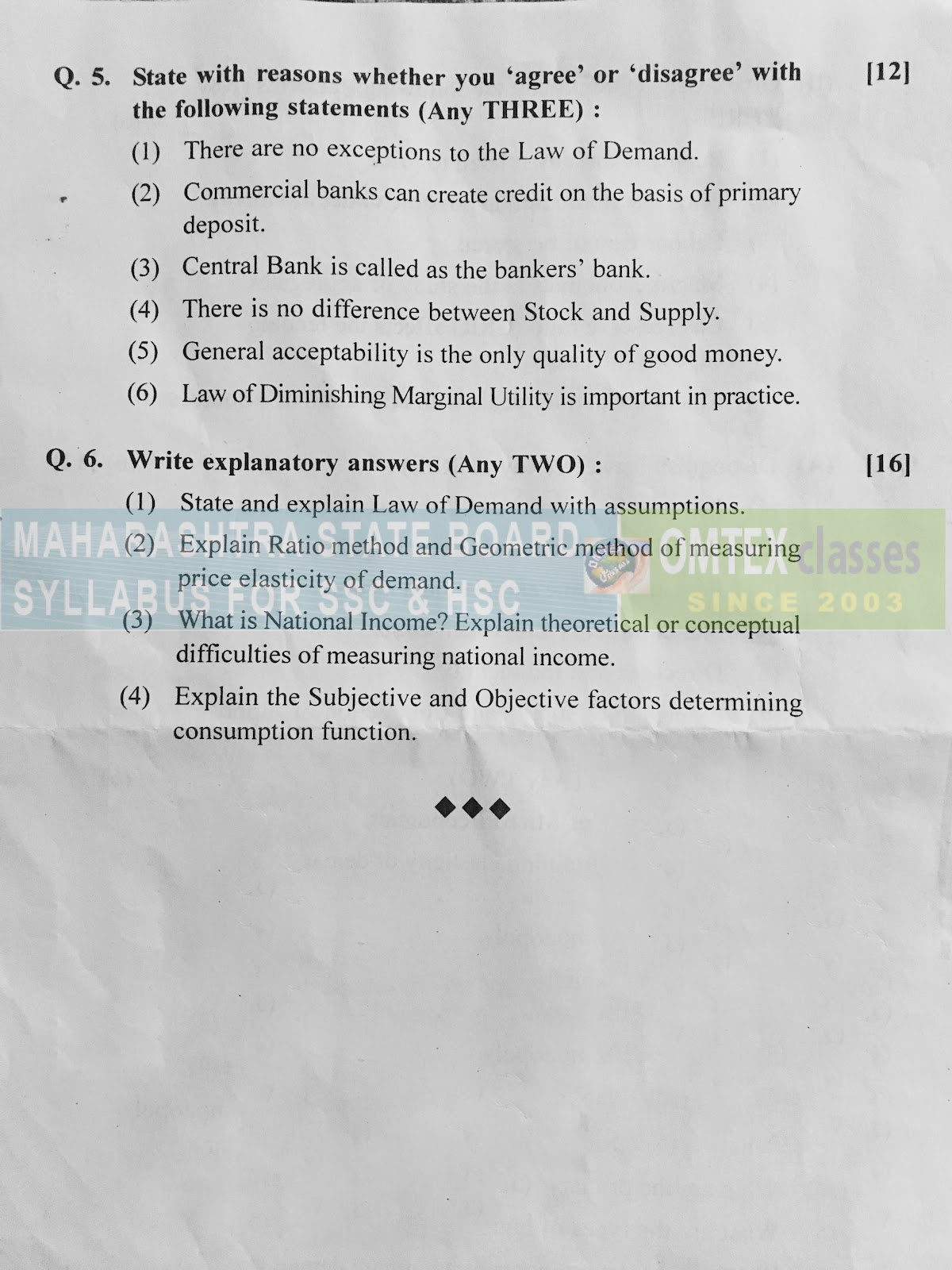SSC BOARD PAPERS IMPORTANT TOPICS COVERED FOR BOARD EXAM 2024

### ECONOMICS HSC MARCH 2017 BOARD PAPERECONOMICS BOARD QUESTION PAPER : MARCH 2017

Note:
(1) All questions are compulsory.
(2) Draw tables/diagrams, wherever necessary.
(3) Figures to the right indicate full marks.
(4) Write answers of every new question on a new page.

Q. 1. A. Fill in the blanks using proper alternatives given in the brackets:  (5)

1.        Market demand is a total demand of all buyers.
2.         Perfectly inelastic demand curve is vertical straight line parallel to ‘OY’ axis.
3.         Other factors remaining constant, when price of a commodity raises there is extension of supply.
4.         National income is flow concept.
5.         Central bank is the apex body of the monetary and banking system of the nation’s economy.

Q1. B. Match the following:  

a.                          Adam Smith - (2) Father of economics.

b.                          Railway  - (3) Public monopoly

c.                          Legal tender money - (7) Fiat money

d.                          D-mat account  - (5) Buying and selling of shares.

e.                          Bank rate  - (6) Quantitative tool of credit control.

Q. 1. C. State whether the following statements are True or False: (6)

1.         Total Revenue = Total quantiry x Price - True.

2.         Demand for necessary goods is inelastic - True.

3.         Capital is a natural factor of production - False.

4.         Consumption expenditure is the only component of aggregate demand - False.

5.         Credit money is created by the central bank of a country. - False

6.         Budget is a monthly statement - False.

Q. 2. (A) Define or explain the following concepts (Any THREE): (6)

Q. 2. B. Give reasons or explain the following (Any THREE): [6 Marks]

Q. 3. A. Distinguish between (Any THREE): (6)

Q.3. B. Write short notes on (Any Two) (6)

Q4. Write short answers for the following questions (Any Three) (12)

Q. 5. State with reasons. Whether you ‘agree’ or ‘disagree’ with the following statements (Any Three) 

Q. 6. Write explanatory answers: (Any two)  

ECONOMICS BOARD PAPERS WITH COMPLETE SOLUTION.
MARCH 2014